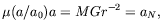2. THE MODIFIED DYNAMICS

This modified dynamics, MOND, introduces a constant with the dimensions of an acceleration, 0, and posits that standard Newtonian dynamics is a good approximation only for accelerations that are much larger than 0. The exact behavior in the opposite limit is described by the specific underlying theory, to be described below. However, the basic point of MOND, from which follow most of the main predictions, can be simply put as follows: a test particle at a distance r from a large mass M is subject to the acceleration a given by(1)

when a << a0, instead of the standard expression a = MGr-2, which holds when a >> a0. The two expressions may be interpolated to give the heuristic relation(2)

where aN is the Newtonian expression for the acceleration, and the interpolating function µ(x) satisfies µ(x)1 when x >> 1, and µ(x)x when x << 1. This expression, while lacking from the formal point of view, is very transparent, and captures the essence of MOND. I shall describe below more presentable theories based on this basic relation, but these are still phenomenological theories into which the form of µ(x) has to be put in by hand. It will hopefully follow one day from a more basic underlying theory for MOND, which we still lack. Most of the implications of MOND do not depend strongly on the exact form of µ. Much of the phenomenology pertinent to the mass discrepancy in galactic systems occurs in the deep-MOND regime (a << a0), anyway, where we know that µ(x)x.